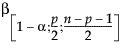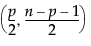wi = 1.0 if Q < K and wi = K/Q otherwise,
where K is a constant equal to the 0.75 quantile of a chi-square distribution with the degrees of freedom equal to the number of columns in the data table, and
where yi = the response for the ith observation, μ = the current estimate of the mean vector, S2 = current estimate of the covariance matrix, and T = the transpose matrix operation. The final step is a bias reduction of the variance matrix.
Spearman’s ρ (rho) Coefficients
Spearman’s ρ correlation coefficient is computed on the ranks of the data using the formula for the Pearson’s correlation previously described.
Kendall’s τb Coefficients
Kendall’s τb coefficients are based on the number of concordant and discordant pairs. A pair of rows for two variables is concordant if they agree in which variable is greater. Otherwise they are discordant, or tied.
 • The sgn(z) is equal to 1 if z>0, 0 if z=0, and –1 if z<0.
 • The ti (the ui) are the number of tied x (respectively y) values in the ith group of tied x (respectively y) values.
 • The n is the number of observations.
 • Kendall’s τb ranges from –1 to 1. If a weight variable is specified, it is ignored.
 • Observations are ranked in order according to the value of the first variable.
 • The observations are then re-ranked according to the values of the second variable.
 •
 • The Ri and Si are ranks of the x and y values.
 • The Qi (sometimes called bivariate ranks) are one plus the number of points that have both x and y values less than the ith points.
 • A point that is tied on its x value or y value, but not on both, contributes 1/2 to Qi if the other value is less than the corresponding value for the ith point. A point tied on both x and y contributes 1/4 to Qi.
When there are no ties among observations, the D statistic has values between –0.5 and 1, with 1 indicating complete dependence. If a weight variable is specified, it is ignored.
where Ri2 is the coefficient of variation from the model regressing the ith explanatory variable on the other explanatory variables. Thus, a large rii indicates that the ith variable is highly correlated with any number of the other variables.
Yi is the data for the ith row
Y is the row of means
n = number of observations
p = number of variables (columns)= (1–αth) quantile of a Betadistribution
n = number of observations
p = number of variables (columns)
Mi = Mahalanobis distance for the ith observation
T2 Distance Measures
The T2 distance is the square of the Mahalanobis distance, so Ti2 = Mi2.
The UCL on the T2 distance is:
n = number of observations
p = number of variables (columns)= (1–αth) quantile of a Betadistribution
Cronbach’s α is defined as
k = the number of items in the scale
c = the average covariance between items
v = the average variance between items
r = the average correlation between items
The larger the overall α coefficient, the more confident you can feel that your items contribute to a reliable scale or test. The coefficient can approach 1.0 if you have many highly correlated items.